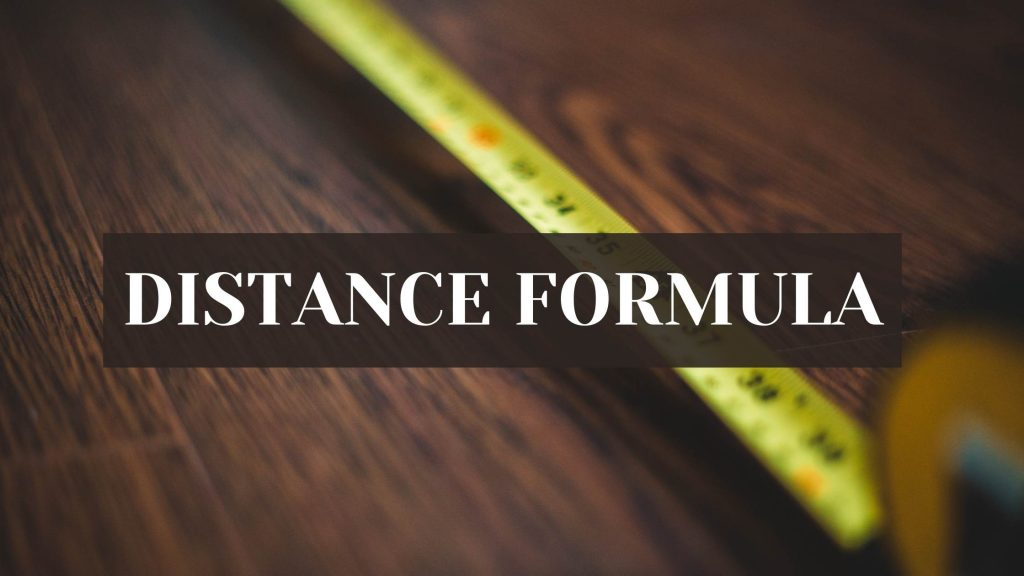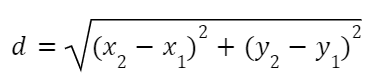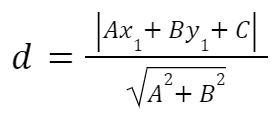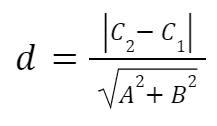Maths
Study Material

# Distance Formula

There are different types of distance formulas in 2D geometry. Distance between two points, distance between a point and a line or distance between two parallel lines etc. Here we will read more about it.

4 minutes longHesap Oluştur

Got stuck on homework? Get your step-by-step solutions from real tutors in minutes! 24/7. Unlimited.

Distance Formula is used to calculate distance magnitude. This distance can be distance between two points, distance between a point and a line or distance between two parallel lines etc, depending on the formula used. Here we will learn about all the distance formulas used in 2D-Geometry.

## What is Distance Formula in 2D?

It is the formula used to measure various distances on the two dimensional plane.

There are different types of distance formulas in 2D geometry.

• Distance between two points
• Distance from a point to a line
• And, Distance between two parallel lines

## Distance Between Two Points

Distance formula used to calculate distance between two given points on a two dimensional plane. Also referred as the Euclidean distance formula.

### Derivation of the Formula

Let us assume two points A(x₁,x₂) and B(y₁,y₂) on the 2D plane.

We will use Pythagoras theorem to derive the formula.

In the ∆ABC, using pythagoras theorem

We can say,

(AB)² = (BC)² + (AC)²

Hence, d² = (x₂ – x₁)² + (y₂ – y₁)²

Therefore, d = √[((x₂ – x₁)² + (y₂ – y₁)²]

### Formula to find Distance Between Two Points## Distance From a Point To a Line

It is the formula used to find the distance of a given point to a line in the 2D plane. The distance is the length of the perpendicular line segment drawn from the point to the given line.

Consider a line L: Ax + By + C = 0 and a point P(x₁,y₁) on the 2D plane. Then the distance(d) from the point(P) to the line(L) is given as:## Distance between Two Parallel Lines

It is the distance between two parallel lines is the shortest distance from a point on one line to a point on other line, i.e It is the perpendicular distance between the two parallel lines.

Let the two lines be  L₁: Ax₁ + By₁ + C1 = 0 and  L₂: Ax₂ + By₂ + C2 = 0, let the perpendicular distance between the two lines be d.

Then the formula for Distance between Two Parallel Lines is given as:## Illustrative Examples on Distance Formula

Example 1: Find the distance between the points (-3, 2), and (4, -3).

Solution: The given two points are (x1,y1) = (-3, 2), and (x2,y2) = (4, -3)

Using the Euclidean distance formula,

Distance = √[(x2−x1)2+(y2−y1)2]

= √[(4+3)2+(-3-2)2]

? √[72+(-5)2] = √(49+25)

= √74

Example 2: Find the distance from the point (2, -3) to the line 3x – 4y = 2.

Solution: The given point is, (x1,y1)= (2, -3).

We can write line equation as 3x – 4y – 2 = 0. Comparing this with Ax + By + C = 0, we get A = 3, B = -4, and C = -2.

Using the distance formula to find the distance from a point to a line,

d = |(3×2)+(-4×-3)+(-2)|/√[32+(-4)2]

=> d = |6+12-2|/√(9+16)

Hence, d = 16/5